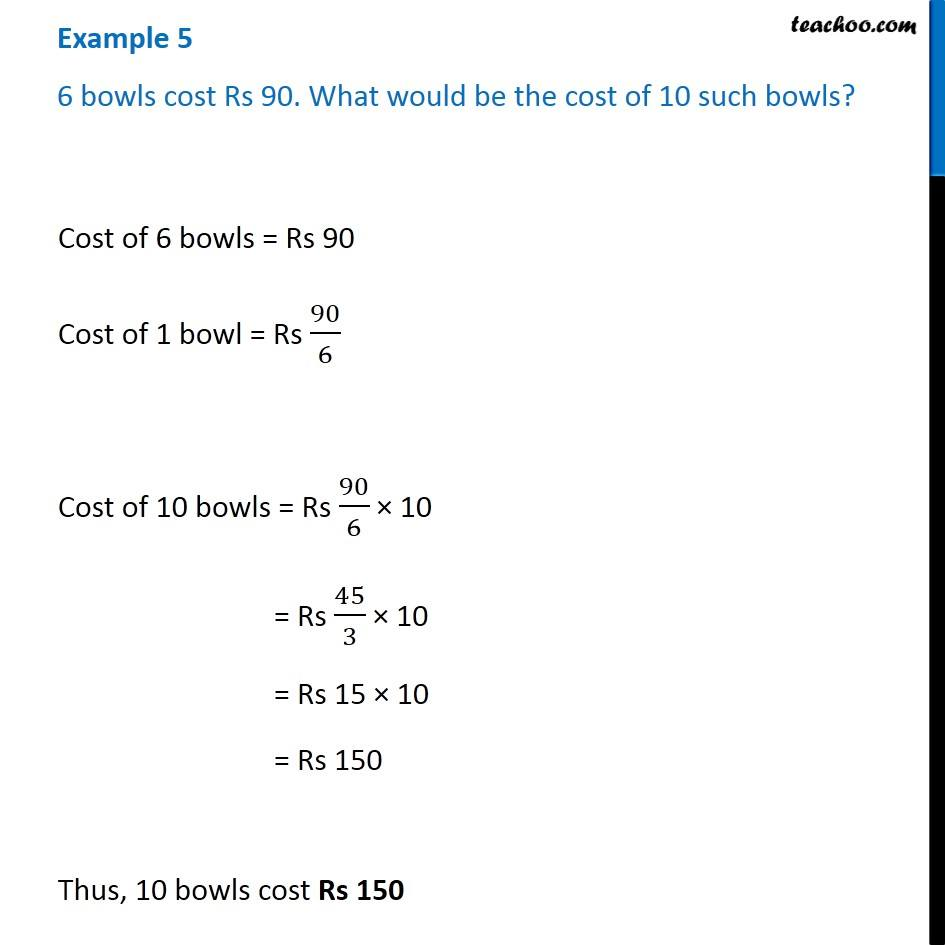Examples

Chapter 8 Class 7 Comparing Quantities
Serial order wise549 people joined Teachoo Black. What are you waiting for?

### Transcript

Example 5 6 bowls cost Rs 90. What would be the cost of 10 such bowls? Cost of 6 bowls = Rs 90 Cost of 1 bowl = Rs 90/6 Cost of 10 bowls = Rs 90/6 × 10 = Rs 45/3 × 10 = Rs 15 × 10 = Rs 150 Thus, 10 bowls cost Rs 150